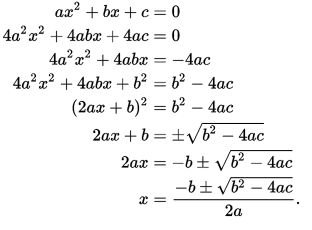The quadratic formula is one of the key formulas a student needs to learn. Without this formula, it is more difficult to solve quadratic equations. When people hear the term “quadratic formula,” they are often nervous because it sounds like a complicated formula. Although it is more advanced than some math topics, it really is not all that difficult if you know the steps. This is one of those formulas you need to be able to memorize.Although it looks really complicated, it is not all that bad once you understand how the formula works.

A standard quadratic equation looks something like this:

+ bx +c = 0

To figure out the problem, you will simply need to plug your numbers into the quadratic formula so you can determine your answer.

In the above problem, a, b, and c must all be known variables and cannot equal 0 or the problem will not be a quadratic equation.

X is the variable and we do not know what this number is yet. The coefficients for the quadratic equation above are: a =5, b =6, c =1 So, the problem will look like this:

+ 6x + 1 = 0

You then need to plug the numbers in the quadratic formula so it looks like this:

x = −6 ± √(62 − 4×5×1)

2×5

Solve for x:

x = 6 ± √(36 − 20)

10

x = −6 ± √(16)

10

x = −6 ± 4

10

x = -1

Once you have mastered the quadratic formula and are successfully using it to solve quadratic equations, it is time to step up your game and begin to learn Graphing With The Quadratic Formula. For this method of solving, you will need graphing paper.

If you had graphed the above problem on graphing paper, you would see this:The curvy lines you draw on the graph are called parabolas. (Funny sounding word, isn’t it!) A parabola should be a smooth, flowing curved line and not one that is segmented with each line being straight from axis to axis.

With practice, using this formula and graphing will become second nature to you and will be beneficial when it comes time to work harder math problems later on. If you find yourself struggling to remember the formula, here is a little song that might help you. You will sing it in the same way you sing “Pop Goes the Weasel.”

It goes a little something like this:

x equals negative b
plus or minus the square root
of b squared minus 4ac
all over 2a

Your best buds might laugh at you if they hear you singing this but it will sure help you remember that pesky formula when it comes time to work out problems in math class or on that hard test!